# 6 Unit Operations and Material Balances

Learning Objectives

By the end of this section, you should be able to:

Define common unit operations and use these when constructing process flow sheets

Recognize the need to apply basic material balances

### Common Units

We will go over four common unit types below, many other units are variants of these basic types of units.

• Mixer: has multiple feed streams and mixes these streams into one output stream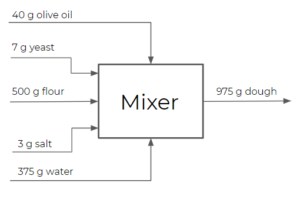• Splitter: a splitter will split a feed stream into multiple streams with the same composition. The exiting streams are not necessarily equal in terms of flow rates, but will always have the same composition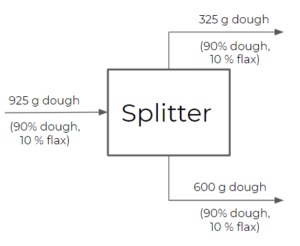• Separator: a separator is similar to a splitter, but has the ability to split streams into different exiting compositions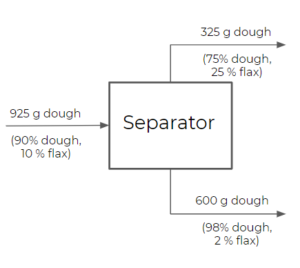• Reactor: a reactor typically consists of a vessel in which a reaction (or multiple reactions) takes place. There are many different types of reactors, some of which you will learn more about in future courses (e.g. CHBE 376 & CHBE 355)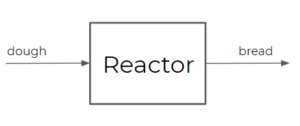Processes are often represented as interconnected process units consisting of mixers, reactors, splitters, and separators among other unit operations.

Examples of other common unit operations:

• Distillation (a separation process using the difference in boiling points of components)
• Absorption (removing components from a vapour stream using a liquid) and stripping (removing components from a liquid stream using a vapour)
• Extraction (a process that purifies a dissolved substance using its solubility in two immiscible liquids)
• Heat exchanger
• Filtration

Example: Vinyl Chloride Production Process

Recall the vinyl chloride production process from the last chapter:

\begin{align*}
C_{2}H_{4} + Cl_{2} → C_{2}H_{4}Cl_{2}&\;\;\;\; (1)\\
C_{2}H_{4}Cl_{2} → C_{2}H_{3}Cl + HCl&\;\;\;\; (2)
\end{align*}

We now consider an additional reaction in the vinyl chloride production process. Most vinyl chloride manufacturers use the combination of direct chlorination and oxychlorination, which is called the balanced process .

\begin{align*}
C_{2}H_{4} + Cl_{2} → C_{2}H_{4}Cl_{2}&\;\;\;\; (1)\;\;\;\; \\
C_{2}H_{4} + 2HCl + \frac{1}{2}O_{2} → C_{2}H_{4}Cl_{2} + H_{2}O&\;\;\;\; (2) \\
C_{2}H_{4}Cl_{2} → C_{2}H_{3}Cl + HCl&\;\;\;\; (3)
\end{align*}

1. Direct Chlorination (last lecture)
2. Oxychlorination
3. Ethylene dichloride pyrolysis (last lecture)

What is the overall reaction and what advantage does this have over what we saw last chapter?

To get the overall reaction, we add all the reactions and cancel any compounds that appear in both the reactants and products of the overall reaction. In the separation process, HCl is recycled back to the oxychlorination reactor if it is not depleted to ensure no HCl is released into the environment. Reaction (1) is balanced with the rest of the process in a way to provide the source of $C_{2}H_{4}Cl_{2}$ for reaction (3). This process gives us only 1 by-product: water. Using the balanced process gives us water as a by-product as opposed to hydrogen chloride.

$2C_{2}H_{4}+Cl_{2}+\frac{1}{2}O_{2} → 2C_{2}H_{4}Cl_{2} + H_{2}O$

This process is carried out in multiple unit operations. To achieve the maximum efficiency and avoid releasing hazardous chemicals, hydrogen chloride produced in reaction 3 is recycled back to the oxychlorination reactor and used reaction (2), as well as unreacted EDC(ethylene dichloride, $C_{2}H_{4}Cl_{2}$) in reaction 3 is recycled to react again.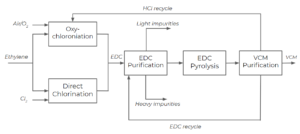• Ethylene enters the process and is split into 2 streams: 1 stream goes to the oxychlorination reactor an the other stream goes to the direct chlorination reactor
• Air and/or oxygen are fed into the oxychlorination reactor and chlorine is fed into the direct chlorination reactor
• EDC produced by both the oxychlorination and direct chlorination processes, and is fed into a purification unit to separate from the other components in the system
• EDC is then fed into the EDC pyrolysis reactor, followed by the VCM(vinyl chloride monomer, $C_{2}H_{3}Cl$) purification unit, which separates HCl, VCM, and EDC into 3 separate streams
Compound Boiling Point ($^{\circ}C$)
$C_{2}H_{4}$ -103.7
$HCl$ -85.05
$Cl_{2}$ -34.04
$C_{2}H_{3}Cl$ -13.4
$C_{2}H_{4}Cl_{2}$ 83.47
$C_{2}H_{3}Cl_{3}$ 114

### Separation of Components

As depicted above, we have light products (the components that are more volatile than our desired product, EDC), heavy products (the components that are less volatile than our desired product), and a stream of EDC. Let’s look at separating the components after direct chlorination: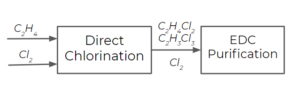For simplicity, say we only have chlorine ($Cl_{2}$), ethylene dichloride (EDC, $C_{2}H_{4}Cl_{2}$), and trichloroethane (TCE, $C_{2}H_{3}Cl_{3}$) produced from a side reaction as products of the direct chlorination step. In reality, there may be other components in the stream, but we will stick to these three as the main components for this analysis. How many streams will come out after the EDC purification processes, and what are the components of each stream? How do we select the temperature for the purification process?

Compound Boiling Point ($^{\circ}C$)
$Cl_{2}$ -34.04
$C_{2}H_{4}Cl_{2}$ 83.47
$C_{2}H_{3}Cl_{3}$ 114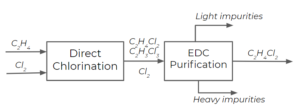From the boiling point temperatures, we can deduce that the light product will be $Cl_{2}$ (boils at a lower temperature) and the heavy product will be $C_{2}H_{3}Cl_{3}$ (TCE) (boils at a higher temperature).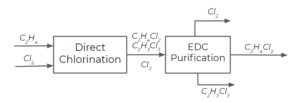We can consider the separation of EDC from TCE and chlorine using 2 distillation columns. In this course, we’ll assume that distillation columns separate a single feed stream into two exit streams. There are more complex column arrangements with a greater number of entering and exit streams, but we won’t go over these in this course. Distillation columns usually operate with a range of temperatures inside them, and we’ll see why this is later in this course. For now, we’ll just assign them an average temperature.

The first column separates EDC and TCE from chlorine by operating at a temperature that is lower than EDC’s boiling temperature, but higher than $Cl_{2}$‘s boiling temperature (let’s say on average $60 ^{\circ}C$):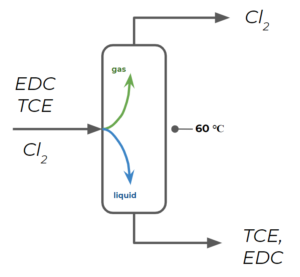The second column separates EDC from TCE by operating at a temperature that is lower than TCE’s boiling temperature, but higher than EDC’s boiling temperature (let’s say, $100 ^{\circ}C$):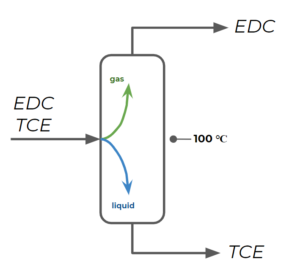Exercise: Separation Process

Consider the following vinyl chloride separation process: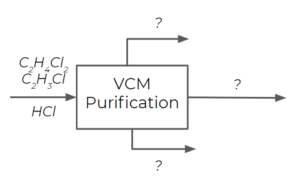The following data is available:

Compound Boiling Point ($^{\circ}C$)
$HCl$ -85.05
$C_{2}H_{3}Cl$ (VC) -13.4
$C_{2}H_{4}Cl_{2}$(EDC) 83.47

How would you set up distillation columns for the separation of vinyl chloride (VC) from hydrogen chloride and EDC? Provide a temperature estimate could these columns be run at for separation.

### Solution

Step 1: Recognize that an easy way to separate 3 compounds is using 2 different distillation columns by separating out EDC in the first column and then separating vinyl chloride from $HCl$ in the second column.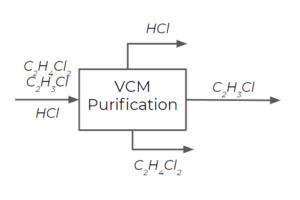Step 2: For the first column, we separate EDC as a liquid, while $HCl$ and vinyl chloride exit the column as gases. We choose a temperature that is lower than the boiling point temperature of EDC, but higher than the boiling point temperature of vinyl chloride. We have a large temperature range that meets these criteria, but we choose $25^{\circ}$ since it is an easy temperature to work with (as it is close to ambient temperatures generally).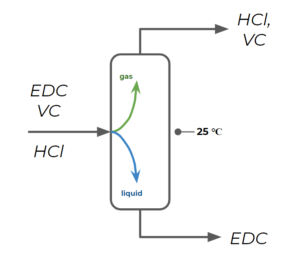Step 3: For the second column, we separate VC as a liquid and HCl as a gas. We choose a temperature that is lower than the boiling point temperature of VC, but higher than the boiling point temperature of HCl. For example, we may choose $-50^{\circ}$ .Note: this is a very cold working temperature for a distillation column and we might try to find ways to change this by manipulating pressure, but we will explore this later in this course and you will learn more through other courses.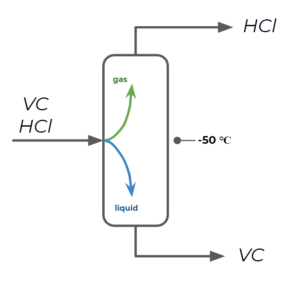## Material Balances

General material balance rules:

• What goes in must come out (or react)
• Total mass into and out of a unit should always be equal
• Molecules or moles may not be conserved, but the atoms should be

We won’t cover material balances in-depth in this course. Instead, I will rely on you applying the concepts you are learning in your Material and Energy Balances course.